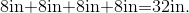## Example Questions

### Example Question #4 : How To Find The Perimeter Of A Square

A square garden has an area of 64 square feet. If you add 3 feet to each side, what is the new perimeter of the garden?

121

25

32

20

44

44

Explanation:

By finding the square root of the area of the garden, you find the length of one side, which is 8. We add 3 feet to this, giving us 11, then multiply this by 4 to get 44 feet for the perimeter.

### Example Question #5 : How To Find The Perimeter Of A Square

The area of the shaded region of a square is 18. What is the perimeter of the square?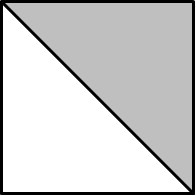28

24

36

20

24

Explanation:

The area of the shaded region, which covers half of the square is 18 meaning that the total area of the square is 18 x 2, or 36. The area of a square is equal to the length of one side squared. Since the square root of 36 is 6, the length of 1 side is 6. The perimeter is the length of 1 side times 4 or 6 x 4.

### Example Question #6 : How To Find The Perimeter Of A Square

The area of a square is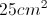.  If the square is enlarged by a factor of 2, what is the perimeter of the new square?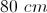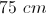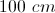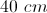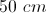Explanation:

The area of a square is given by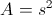so we know the side is 5 cm.  Enlarging by a factor of two makes the new side 10 cm.  The perimeter is given by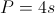, so the perimeter of the new square is 40 cm.

### Example Question #7 : How To Find The Perimeter Of A Square

The diagonal of a square has a length of 10 inches. What is the perimeter of the square in inches squared?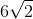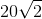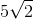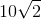Explanation:

Using the Pythagorean Theorem, we can find the edge of a side to be √50, by 2a2=102. This can be reduced to 5√2. This can then be multiplied by 4 to find the perimeter.

### Example Question #8 : How To Find The Perimeter Of A Square

What is the perimeter of a square with an area of?Explanation:

1. Find the side lengths: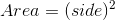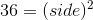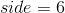2. Use the side lengths to find the perimeter: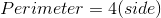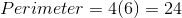### Example Question #1 : How To Find The Perimeter Of A Square

Find the perimeter of a square whose area is.Explanation:

To solve, you must first find the side length.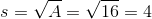Then, you must multiply the side length by 4 since there are 4 sides. Thus,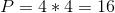In this case, volume and perimeter were the same numerical value, but this won't always be the case.

### Example Question #2 : How To Find The Perimeter Of A Square

Find the perimeter of a square with side length.Explanation:

To find perimeter, simply multiply side length by. Thus,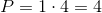### Example Question #3 : How To Find The Perimeter Of A Square

The area of a square is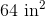, what is the perimeter of the square?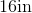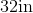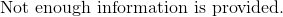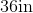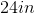Since the sides of a square are all the same, the area of a square can be found by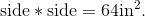Therefore, the side of the square must be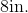The perimeter of a square can be found by adding up all of the four sides: